# Angle in Standard Position

Brigette Banaszak, Michael Quist
• Author
Brigette Banaszak

Brigette has a BS in Elementary Education and an MS in Gifted and Talented Education, both from the University of Wisconsin. She has taught math in both elementary and middle school, and is certified to teach grades K-8.

• Instructor
Michael Quist

Michael has taught college-level mathematics and sociology; high school math, history, science, and speech/drama; and has a doctorate in education.

Learn what an angle in a standard position is. Learn how to draw an angle in a standard position, practice using degrees and radians, and study examples of the same. Updated: 01/02/2022

Show

## What Is Standard Position?

Angles have the same measure regardless of their orientation. In the triangle, each of the three angles has a measure of 60 degrees even though they all have different orientations.Angular geometry refers to the size of an angle rotated around a circle from a starting point. That starting point is called standard position. What is an angle in a standard position? On the Cartesian plane, an angle in standard position has a vertex at the origin, which intersects x- and y-axes, and the first side extends to the right along the x-axis. On the Cartesian plane, the first side between Quadrants I and IV is called the initial side. For an example, see the diagram given below. The other side of the angle is called the terminal side.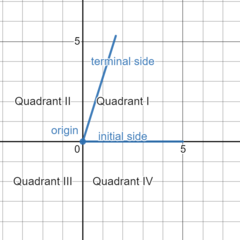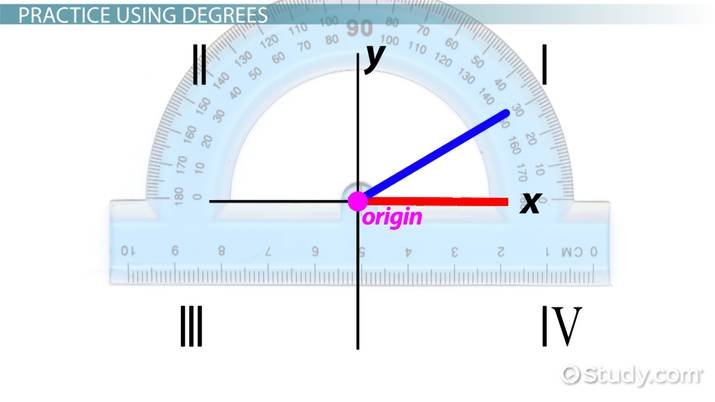An error occurred trying to load this video.

Try refreshing the page, or contact customer support.

Coming up next: Coterminal Angles: Definition & Examples

### You're on a roll. Keep up the good work!

Replay
Your next lesson will play in 10 seconds
• 0:00 Angle Positions
• 1:03 Standard Position for Angles
• 1:47 Practice Using Degrees
• 4:29 Lesson Summary
Save Save

Want to watch this again later?

Timeline
Autoplay
Autoplay
Speed Speed

## How to Draw an Angle in Standard Position

How does someone draw an angle in a standard position? A protractor will be needed for this.To draw an angle in standard position, follow these steps:

1. Start by placing the baseline of a protractor on a Cartesian plane with the vertex at the origin.
2. Trace the first or initial side of the angle along the horizontal x-axis to the right.
3. Find the desired degree measurement on the protractor, and mark that spot.
4. Draw the second side, or terminal side, of the angle extending from the vertex to the marked point.

Depending on the size of the angle, the terminal side can be in any four quadrants of the Cartesian plane. A 360 degrees rotation makes a full circle. An angle whose terminal side lies along one axis is known as a quadrantal angle. A quadrantal angle whose terminal side lies straight up along the y-axis, for example, is a 90-degree angle. A quadrantal angle whose terminal side lies along the x-axis extending left of the origin is a 180-degree angle.

Angles in standard position can be considered positive or negative. A positive angle in a standard position is measured in a counterclockwise direction from the initial side to the terminal side. A negative angle in standard position is measured in a clockwise direction from the initial side to the terminal side.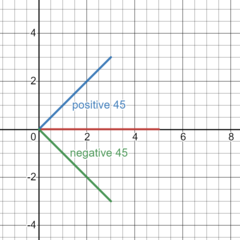### Coterminal and Reference Angles

Coterminal angles are standard positions with the same terminal side. The angles shown in the diagram, +135 and -225, are coterminal.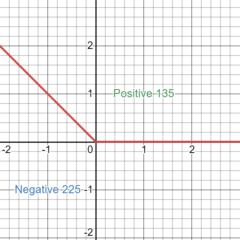A reference angle is the smallest angle that can be drawn between the terminal side of an angle and the x-axis. The diagram shows a 135-degree angle and its 60-degree reference angle.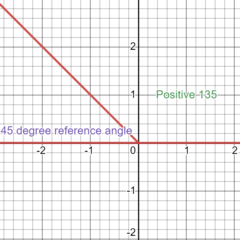Reference angles can be used to find the trigonometric functions sine and cosine of the original angle, based on what quadrant the terminal side of the original angle lies in.

## Examples of Drawing Angles in Standard Position

When drawing angles in standard position, follow the steps:

1. Start by placing the baseline of a protractor on a Cartesian plane with the vertex at the origin.
2. Trace the first or initial side of the angle along the horizontal x-axis to the right.
3. Find the desired degree measurement on the protractor, and mark that spot.
4. Draw the second side, or terminal side, of the angle extending from the vertex to the marked point.

Here are some examples of angles drawn in standard position.

#### Example 1

Draw a 90-degree angle in a standard position.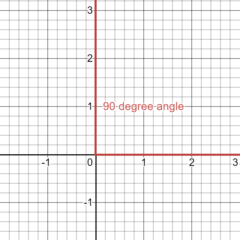It is a positive angle, so it is measured in a counterclockwise direction from the initial side to the terminal side. The terminal side of this angle lies along the y-axis. It is a quadrantal angle.

#### Example 2

Draw a 210-degree angle in a standard position.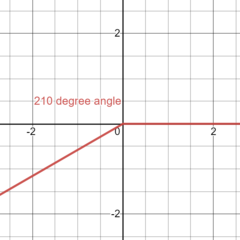It is a positive angle, so it is measured in a counterclockwise direction from the initial side to the terminal side. The terminal side of this angle lies in Quadrant III.

#### Example 3

Draw a -45 degree angle in standard position.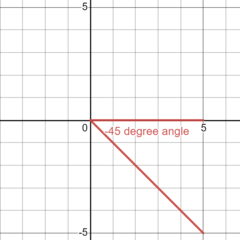It is a negative angle, so it is measured in a clockwise direction from the initial side to the terminal side. The terminal side of this angle lies in Quadrant IV.

## Measuring Angles in Standard Position with Degrees vs. Radians

Angles can be measured in degrees or radians. A radian is a unit of measure for angles based on the fact that the ratio of the circumference of a circle to the diameter of the circle is equal to the constant pi, or {eq}\pi {/eq}

An angle in standard form using radians is based on a unit circle, a circle with a center at the origin and a radius of 1. The diameter of a unit circle is twice the radius, or 2, so its circumference is {eq}2\pi {/eq}. Angle measurements in radians are based on the circumference of the unit circle, {eq}2\pi {/eq}.

• A full circle, or 360 degrees, is equal to {eq}2\pi {/eq} radians, which means
• 180 degrees equals {eq}\pi {/eq} radians, and
• 90 degrees equals {eq}\frac{\pi}{2} {/eq} radians.

To unlock this lesson you must be a Study.com Member.

#### Why is standard position important in mathematics?

Angles in standard position are used in mathematics in geometry, trigonometry, and calculus. They are also used in real-life to calculate angular speed.

#### How to find the standard position of an angle?

An angle in the standard position on a Cartesian plane has its vertex at the origin. One side, the initial side, lies along the x-axis to the right. The other side is referred to as the terminal side.

#### What is a reference angle in standard position?

A reference angle is the smallest angle that can be drawn between the terminal side of an angle and the x-axis. For example, the reference angle of a 120-degree angle will be 60-degree.

#### What does it mean to draw an angle in standard position?

An angle in standard position on a Cartesian plane has its vertex at the origin, and its initial side lies along the x-axis. The other side of the angle is called the terminal side.

### Register to view this lesson

Are you a student or a teacher?